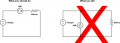Input Impedance of a milliameter.

GiannisMandelos

Joined Jan 19, 2019
29
Hi everyone! I would like to ask what does the Input Impedance of a digital milliameter mean? Does it refer to the meter's power supply circuit? And if so, what does it tell on how to apply my power supply on it? I ordered a 500 mAmeter and it's specifications refered the following: " Measure the impedance: 1Ω", while other milliameters just stated: "Input Impedance= 10mohms, 1ohm etc". Should I use any load at my power supply circuit, like a voltage divider through two series resistors? Thank you for your tiime!

MrChips

Joined Oct 2, 2009
25,040
Hi everyone! I would like to ask what does the Input Impedance of a digital milliameter mean? Does it refer to the meter's power supply circuit? And if so, what does it tell on how to apply my power supply on it? I ordered a 500 mAmeter and it's specifications refered the following: " Measure the impedance: 1Ω", while other milliameters just stated: "Input Impedance= 10mohms, 1ohm etc". Should I use any load at my power supply circuit, like a voltage divider through two series resistors? Thank you for your tiime!
Knowledge of the resistance (called burden resistance) of every measuring instrument is important.

1) An ideal voltmeter would have infinite input resistance. That is, it should take zero current from the circuit under test.
2) An ideal current meter would have zero burden resistance. That is, the voltage across the meter would be zero volts.

Current meters are never placed across a voltage source. If you do you likely will destroy the meter or blow the internal protection fuse. Current meters are always placed in series with your circuit. In other words, you must break the circuit and insert the meter to bridge the break. Current meters are often designed to be used with a shunt resistor which becomes the low resistance bridge. You want the resistance to be as low as possible. Hence the resistance of a current meter is often in the mohms range, i.e. less than 1 ohm.No, you do not use a voltage divider when using a current meter.

GiannisMandelos

Joined Jan 19, 2019
29
Thank you for your answer but I think I did not asked my question correctly. I refered to the meter's power supply circuit and not the circuit subjected to the meter's measurement. I mean, every meter need it's own power sypply in order to work and that's exactly what I am talking about. Does the Input Impedance refer's to the meter's power supply circuit (I think it does)? This specific milliameter needs a 5 Volt power supply. Should I supply it using an isolated converter that has an unregulated 12V output, through a voltage divider that drops my voltage down to 5 Volt? Because if the Input Impedance of 1 ohm is referd to the meter's power supply circuit, I think that I cant supply it that way. Last, I have noticed some milliameters that their specifications stated "Input Impedance= 10ΜΩ, or even bigger".

crutschow

Joined Mar 14, 2008
28,549
I have noticed some milliameters that their specifications stated "Input Impedance= 10ΜΩ, or even bigger".
That's a specification for a voltmeter, not an ammeter.

•GiannisMandelos

ericgibbs

Joined Jan 29, 2010
14,702
hi GM,
Note the m or M [ small or big ] is important.

For an Ammeter you may see 10mOhm = 0.01 Ohm and for a voltmeter 10M Ohm = 10,000,000 Ohms.
ie: m= milli and M = Mega

Do you need any more help.?
E

•GiannisMandelos

MrChips

Joined Oct 2, 2009
25,040
•